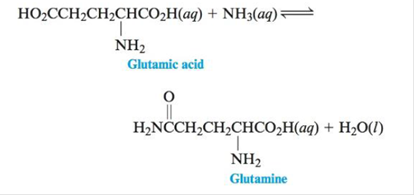# One reaction that occurs in human metabolism is For this reaction ∆G° = 14 kJ at 25°c. a. Calculate K for this reaction at 25°C. b. In a living cell this reaction is coupled with the hydrolysis of ATP. (See Exercise 75.) Calculate ∆G° and K at 25°C for the following reaction: Glutamic acid ( a q ) + ATP ( a q ) + NH 3 ( a q ) ⇌ Glutamine ( a q ) + ADP ( a q ) + H 2 PO 4 − ( a q )### Chemistry: An Atoms First Approach

2nd Edition
Steven S. Zumdahl + 1 other
Publisher: Cengage Learning
ISBN: 9781305079243

#### Solutions

Chapter
Section### Chemistry: An Atoms First Approach

2nd Edition
Steven S. Zumdahl + 1 other
Publisher: Cengage Learning
ISBN: 9781305079243
Chapter 16, Problem 76E
Textbook Problem
17 views

## One reaction that occurs in human metabolism isFor this reaction ∆G°= 14 kJ at 25°c.a. Calculate K for this reaction at 25°C.b. In a living cell this reaction is coupled with the hydrolysis of ATP. (See Exercise 75.) Calculate ∆G° and K at 25°C for the following reaction: Glutamic acid ( a q ) +   ATP ( a q ) + NH 3 ( a q ) ⇌ Glutamine ( a q ) +   ADP ( a q ) + H 2 PO 4 − ( a q )

(a)

Interpretation Introduction

Interpretation: One of the reactions of human metabolism and its ΔG° value is given. The value of K is to be calculated at the given temperature. The coupling of the stated reaction with the reaction corresponding to the hydrolysis of ATP is given. The value of ΔG° and K for this reaction at given temperature is to be calculated.

Concept introduction: Equilibrium constant K , is defined as the ratio of the concentration of products to that of the reactants at equilibrium. If a given reaction is at equilibrium, then the free energy change will be,

ΔG=0Q=K

ΔG°=RTln(K)

### Explanation of Solution

Given

The value of ΔG° for the reaction of human metabolism is 14kJ .

Given temperature is 25°C .

The conversion of degree Celsius (°C) into Kelvin (K) is done as,

T(K)=T(°C)+273

Hence,

The conversion of 25°C into Kelvin is,

T(K)=T(°C)+273T(K)=(25+273)K=298K

Formula

ΔG°=RTln(K)</

(b)

Interpretation Introduction

Interpretation: One of the reactions of human metabolism and its ΔG° value is given. The value of K is to be calculated at the given temperature. The coupling of the stated reaction with the reaction corresponding to the hydrolysis of ATP is given. The value of ΔG° and K for this reaction at given temperature is to be calculated.

Concept introduction: Equilibrium constant K , is defined as the ratio of the concentration of products to that of the reactants at equilibrium. If a given reaction is at equilibrium, then the free energy change will be,

ΔG=0Q=K

ΔG°=RTln(K)

### Still sussing out bartleby?

Check out a sample textbook solution.

See a sample solution

#### The Solution to Your Study Problems

Bartleby provides explanations to thousands of textbook problems written by our experts, many with advanced degrees!

Get Started

Find more solutions based on key concepts
Two tablespoons of peanut butter offer about the same amount of protein as a hot dog. T F

Nutrition: Concepts and Controversies - Standalone book (MindTap Course List)

How long does it take light to cross the diameter of our Milky Way Galaxy?

Horizons: Exploring the Universe (MindTap Course List)

Does the cell cycle refer to mitosis as well as meiosis?

Human Heredity: Principles and Issues (MindTap Course List)

An ideal gas is enclosed in a cylinder with a movable piston on top of it. The piston has a mass of 8 000 g and...

Physics for Scientists and Engineers, Technology Update (No access codes included)